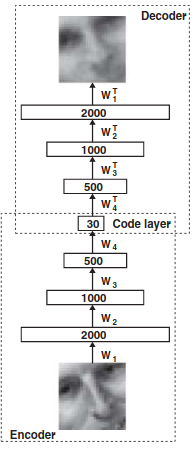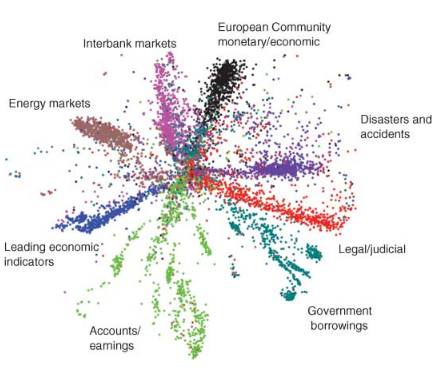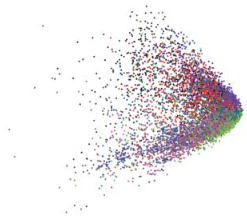Reducing the dimensionality of data has many valuable potential uses. The low-dimensional version of the data can be used for visualisation, or for further processing in a modelling pipeline. The low-dimensional version should capture only the salient features of the data, and can indeed be seen as a form of compression. Many techniques for dimensionality reduction exists, including PCA (and its kernelized variant Kernel PCA), Locally Linear Embedding, ISOMAP, UMAP, Linear Discriminant Analysis, and t-SNE. Some of these are linear methods, while others are non-linear methods. Many of the non-linear methods falls into a class of algorithms known as manifold learning algorithms.

## Architecture

The dimensionality reduction technique discussed in this paper is based on neural networks, and is known as the autoencoder. An autoencoder is essentially a non-linear generalisation of PCA. The autoencoder architecture consists of an encoder network and decoder network, with a latent code bottleneck layer in the middle (see below figure). The goal of the encoder is to compress the input vector into a low-dimensional code that captures the salient features / information in the data. The goal of the decoder is to use that code to reconstruct an approximation of the input vector. The two networks are parameterised as multi-layer perceptrons (MLPs), and the full autoencoder (encoder + decoder) is trained end-to-end using gradient descent. Formally, the goal of an autoencoder is to minimise $L(x, g(f(x)))$, where $L$ is some loss function, $f$ is the encoder network, and $g$ is the decoder network.## Pre-Training

One important trick performed in the paper is pre-training of the autoencoder. This is done in order to get the weights of the network to be at a suitable initialisation such that fine-tuning is easier and more effective. The pre-training is done in a greedy, layer-wise manner (i.e. each pair of layers is pre-trained separately). This pre-training is done using a restricted Boltzmann machine (RBM).

## Results

It is important to recall that an autoencoder is performing non-linear dimensionality reduction, and as such should learn a better low-dimensional data manifold than linear methods such as PCA or factor analysis. We can see a comparison between the low-dimensional representations learned by LSA and an autoencoder in the below figure (applied to documents). Clearly, the autoencoder appears to learn a better representation.